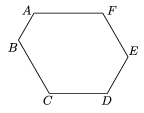Mathematical and Physical Journal
for High Schools
Issued by the MATFUND Foundation
 Already signed up? New to KöMaL?

# New exercises and problems in MathematicsFebruary 2005## New exercises for beginners

Solutions can be submitted only by students of grade 9. Maximum score for each exercise (sign "K") is 6 points.

K. 31. Each interior angle of the hexagon in the figure is 120o. Prove that

AB + FA = CD + DE.K. 32. Three children select books in the following way out of five different books in a library. One of each book is lying on a table, and every child puts his reader's card on the top of the book that he would like to borrow. (The same kind of book may be borrowed by more than one reader, in that case there will be more than one cards on the book. On the other hand, each child is only allowed to borrow one book.) Two arrangements of the cards are considered different if there is at least one child who marked different books in the two arrangements. How many possible arrangements are there altogether?

K. 33. A little boy has got 11 plastic cylinders. If he orders them by increasing height, each of them is 2 cm taller than the previous one (standing on their bases). The last cylinder in the row is exactly as tall as the middle one and one of its neighbours on top of each other. What is the height of the column that he can build by placing all the 11 cylinders on top of each other?

K. 34. Solve the following equation:

(x2-4)2 (x+3)2+ (x+2)2(x-9)2=0.

K. 35. The medians of a triangle are known to halve its area and to be concurrent. Is it true that the lines that halve the area and are parallel to a side also pass through the centroid of the triangle?

K. 36. How many integers of at least two digits are there that are less than six million and for which the preceding number and the following number are both palindrome numbers? What are these integers? (A whole number is called a palindrome number if its digits listed in the opposite order form the same number.)## New exercises

Maximum score for each exercise (sign "C") is 5 points.

C. 795. The number $\displaystyle \overline{a_1a_2a_3a_4a_5a_6}$ in decimal form is divisible by 7. Prove that $\displaystyle \overline{a_6a_1a_2a_3a_4a_5}$ is also divisible by 7.

C. 796. The length of the radius of the incircle of a right-angled triangle is 0.45 times that of the altitude drawn to the hypotenuse. Find the measures of the acute angles.

C. 797. The legs of a square dining table are 70 cm, 71 cm, 72.5 cm and 72 cm long when measured counterclockwise. Will this table be rocking, that is are there two legs that never touch the floor at the same time?

C. 798. Solve the following equation:

3.4x+(3x-10)2x+3-x=0.

C. 799. There are ants sitting at the vertices of a tetrahedron, one at each. At a given moment the ants start crawling along a randomly chosen edge to a neighbouring vertex. What is the probability that two ants will meet on an edge or at a vertex?## New problems

The maximum scores for problems (sign "B") depend on the difficulty. It is allowed to send solutions for any number of problems, but your score will be computed from the 6 largest score in each month.

B. 3792. Rearranging the digits of the positive integer M we obtain the positive integer N. (Leading zeros are not permitted). Is it possible to have two different positive integer powers of 2 this way? (4 points)

B. 3793. P is a point inside the square ABCD such that AP = 1, BP = 2, CP = 3. Find the distance DP. (3 points)

B. 3794. Prove that if 0<q<p<1 then p+3q<1+4pq. (3 points)

B. 3795. Given are in the plane two points whose distance is 1 unit. Construct, with a pair of compasses only, two points whose distance is equal to $\displaystyle \sqrt{2}$. (5 points)

B. 3796. Two tangents are drawn from the exterior point A to the circle k. The points of tangency are E and F, the midpoint of the line segment EF is G. A line through A meets the circle at the points B and C. Prove that EF bisects the angle BGC. (4 points)

B. 3797. The triangle ABC is equilateral. What is the locus of the points P inside the triangle whose distance from the side AB is equal to the geometric mean of its distances from the sides BC and CA? (4 points)

B. 3798. Let $\displaystyle \alpha=2+\sqrt3$. Prove that [$\displaystyle \alpha$n]=$\displaystyle \alpha$n+ alpha-n-1 for every positive integer n. (4 points)

B. 3799. The intersection of two congruent equilateral triangles is a hexagon. Prove that the sum of the lengths of every other side of this hexagon is equal to that of the remaining three sides. (4 points)

B. 3800. There is an ant sitting at each vertex of a cube. At a given time instant, each ant starts crawling along an edge chosen at random to another vertex. What is the probability that two ants will meet on or at the end of their ways? (4 points)

B. 3801. Let P(n) denote the sum of the first n prime numbers. Prove that there is a square number between P(n) and P(n + 1). (5 points)Maximum score for each advanced problem (sign "A") is 5 points.

A. 365. Let

$\displaystyle a_n=\sqrt[n]{(n+1)!}-\sqrt[n]{n!}\,.$

Show that an$\displaystyle \ge$1 for all n, and the sequence an is not bounded.

A. 366. Let p(n) be the number of partitions of n, and let r(n) denote the number of those partitions in which n is equal to the sum of different odd numbers. Prove that p(n) and r(n) are of the same parity.

A. 367. a) D is an interior point of the acute-angled triangle ABC, such that

Find the locus of the points D. bE is an interior point of the acute-angled triangle ABC, such that

AB.BC.CA=AE2.BC+BE2.CA+CE2.AB.

Find the locus of the points E. (Based on the idea of S. Dobos)